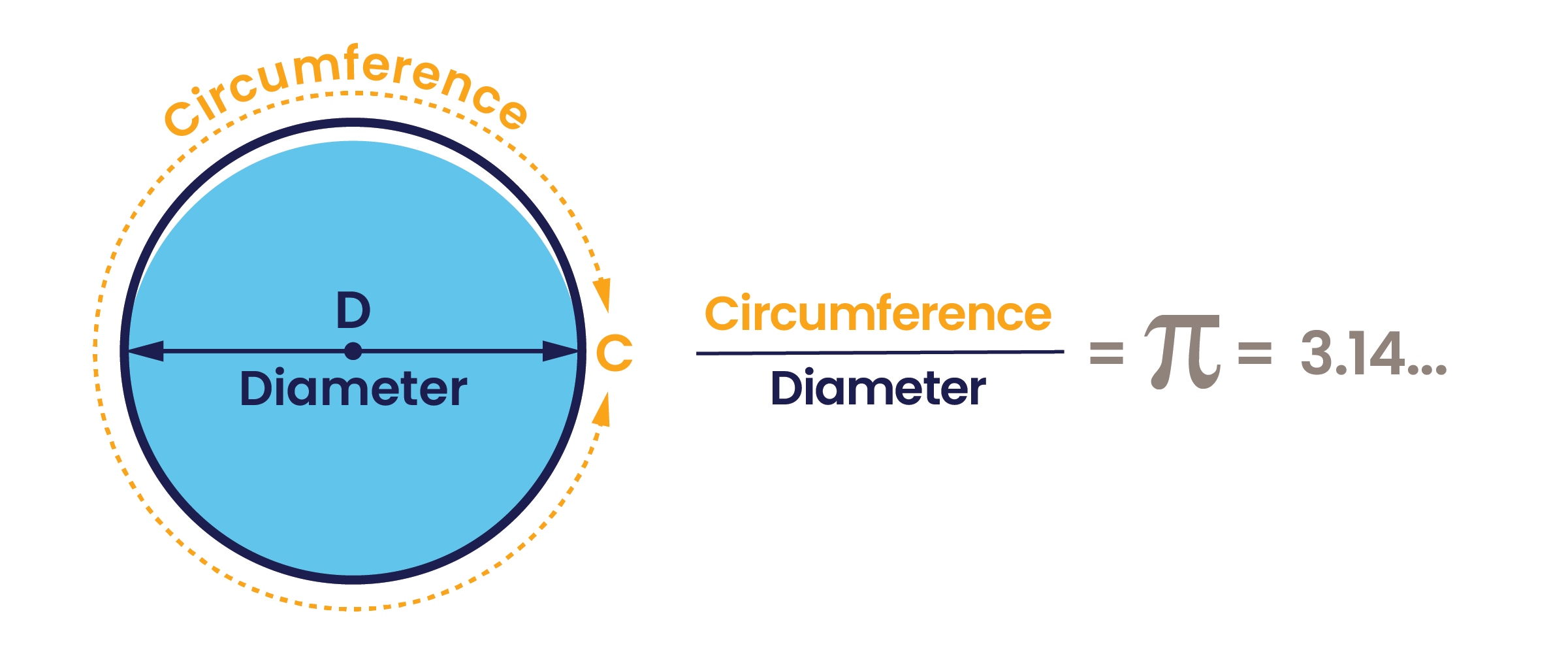## The power of piMarch 14, or 3/14, is Pi Day and is celebrated around the world in honor of the well-known mathematical constant that starts with 3.14 and is the ratio of any circle’s circumference to its diameter. In layman’s terms, it means that for any circle, the distance around the edge is a little more than three times the distance across that circle.

At CAP, pi is more than just a memorable number or fun day to eat pie. In fact, pi — often represented by the lower-case Greek letter π– is extremely important to CAP because we typically use circular shaped pipelines to both move water and measure the amount being pumped.To accurately measure water, we need to know how much is delivered. Here’s where the math comes in.

First, we need to determine flow volume, which requires two numbers, velocity (V) and area (A).
Flowmeters measure how fast water is passing through a single point over time and provides us with the velocity, or V, for our equation.
Determining the area, or A, of the circle at the point where the water is crossing is a simple equation using pi. A = π * r2 , where r = the radius of the circle (half the diameter).

Once you have those two numbers, you can use equation to determine flow rate, or Q
Q = Velocity x Area or Q = V*A

So, let’s say Mark Wilmer Pumping Plant was operating three of the six pumps over a 24-hour period, and the flowmeter was indicating water velocity at 17 feet/second.  One of the two discharge pipes is in service and the inside diameter measures 12 feet.

Flow rate would be Q = (17 ft/sec) * (π) * (6 ft)2 = 1,922 cubic feet per second (ft3/sec).

Since 24 hours is 86,400 seconds, then 1,922 ft3/sec * 86,400 sec =  166 million ft3 of water.  That converts to about 3,812 acre-feet over the 24 hour period.

Water calculations made easier thanks to pi. Happy Pi Day!